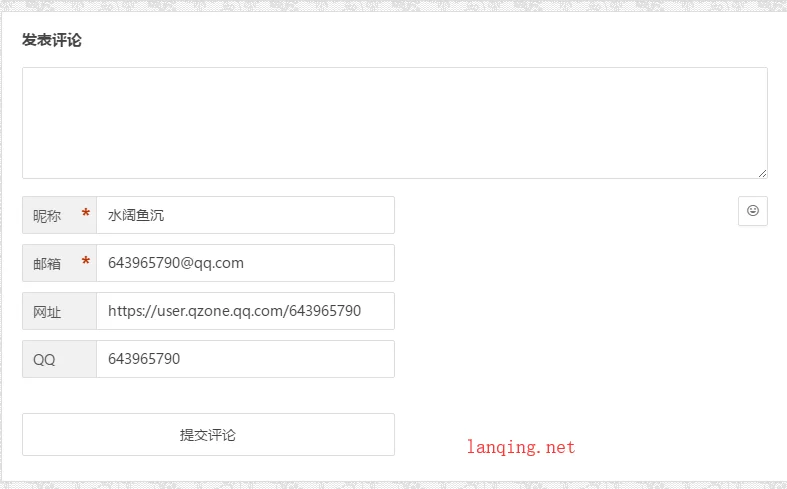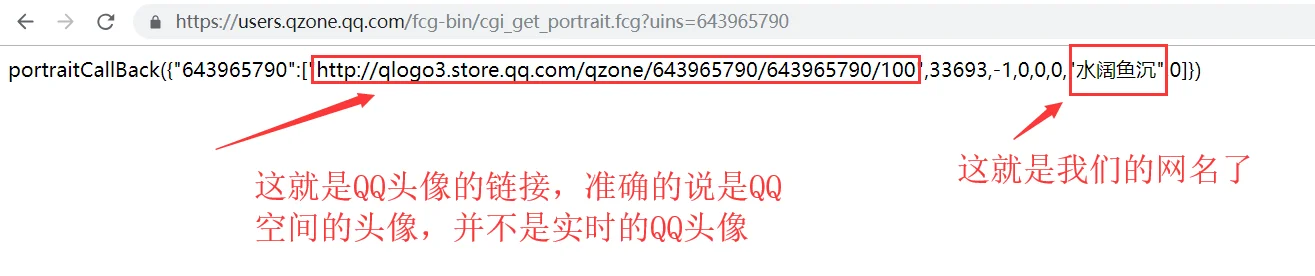# 修复知更鸟Begin主题的QQ获取信息填充功能```<?php
error_reporting(0);
\$table_name = \$wpdb->prefix . "qq_usermeta";
if (\$_SERVER['REQUEST_METHOD'] == 'POST' && isset(\$_POST['type'])) {
if (\$_POST['type'] == "qq") {
if (isset(\$_POST['qq']) && \$_POST['qq'] == "") {
\$message = "请输入QQ";
\$status = "1";
} else {
\$qq = \$_POST['qq'];
\$status = "0";
}
} elseif (\$_POST['type'] == "checkemail") {
\$qq = \$_POST['qq'];
\$email = \$_POST['email'];
\$status = "0";
} else {
}
} else {
\$message = "请求方式错误";
\$status = "1";
}
//echo \$qq;
//这里就是我修改过的，主要是切割取参
\$nameurl = "http://users.qzone.qq.com/fcg-bin/cgi_get_portrait.fcg?uins=" . \$qq;
\$ch = curl_init();
\$timeout = 5;
curl_setopt(\$ch, CURLOPT_URL, \$nameurl);
curl_setopt(\$ch, CURLOPT_RETURNTRANSFER, 1);
curl_setopt(\$ch, CURLOPT_CONNECTTIMEOUT, \$timeout);
\$contents = curl_exec(\$ch);
curl_close(\$ch);
\$info = iconv('GBK', 'UTF-8', \$contents);
\$strarr = explode(',', \$info);
\$req = explode('"', \$strarr);
\$touxiang1 = explode('[', \$strarr);
\$touxiang2 = explode('"', \$touxiang1);
if (\$_POST['type'] == "qq") {
if (count(\$results) == "0") {
\$email = \$qq . "@qq.com";
\$url = "https://user.qzone.qq.com/" . \$qq;
} else {
\$email = \$results[email];
\$url = ',"url":"' . \$results . '"';
}
}
\$logourl = \$touxiang2;
if (\$_POST['type'] == "qq") {
if (\$status == "0") {
print <<<END
END;
} else {
print <<<END
{"message":"{\$message}","status":"{\$status}"}
END;
}
} elseif (\$_POST['type'] == "checkemail") {
if (\$status == "0") {
print <<<END
{"status":"{\$status}"}
END;
} else {
print <<<END
{"message":"{\$message}","status":"{\$status}"}
END;
}
}```

1.谢谢，我试试

QQ